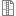# Maybe there is a bug on Handle(Geom_Curve)->value()? Hope I am wrong T.T

Hi Bros, I'm JianBaoxia.

Today, while using Handle(Geom_Curve)->value() to obtain some points on a B-spline curve, I encountered some strange phenomena. Therefore, I couldn't wait to discuss and share with you. To illustrate the problem, I wrote a simple demo, which is as follows:

I read in a B-spline curve with parameter values ranging from 0 to 1. Using Handle(Geom_Curve)->value(), I generated five points on the curve with parameter values of {0, 0.005, 0.5, 0.995, 1}. Then, I used "GeomAPI_ProjectPointOnCurve" to calculate the distance from each of the five points to the curve to determine whether they were located on the curve. The answer was affirmative, as the maximum distance between the points and the curve was at the order of 1e-15, which is far smaller than the tolerance of 1e-7. However, when I outputted the points and the curve together, I found that only the first and last points were truly on the curve, while the middle three had some distance away from the curve. I measured the distances of the middle three points on FreeCAD and obtained the values of 1.2e-1, 4.07e-7, and 8.21e-5, respectively. Clearly, the errors of these three points are all greater than the tolerance of 1e-7.

So, I'm very puzzled as to where the problem lies. According to the formula for NURBS curves, there should be no errors in calculating the points on the curve. Could it be a display issue? Are these five points actually completely on the curve? However, if that were the case, there should be some visible deviation for the first and last points on FreeCAD, but in fact, these two points look completely on the curve.

There is my demo、B-spline curve file: "aEdge.step" and result file: "aSewShape.step":

[CODE]

int main()

{

TopoDS_Edge aEdge;

for (TopExp_Explorer exp(aShape, TopAbs_EDGE); exp.More(); exp.Next())

aEdge = TopoDS::Edge(exp.Current());

Standard_Real _1, _2; // no use

Handle(Geom_Curve) aCur = BRep_Tool::Curve(aEdge, _1, _2);

// sample five points on curve

TColStd_Array1OfReal sampArr(1, 5);

TColgp_Array1OfPnt sampPntsArr(1, 5);

sampArr = 0.;

sampArr = 0.005;

sampArr = 0.5;

sampArr = 0.995;

sampArr = 1.;

for (int i = 1; i <= 5; i++)

{

sampPntsArr = aCur->Value(sampArr);

}

// test whether pnt on curve

for (int i = 1; i <= 5; i++)

{

gp_Pnt pnt = sampPntsArr;

GeomAPI_ProjectPointOnCurve gppc(pnt, aCur); // get the shortest distance bettwen point and curve

if (gppc.LowerDistance() <= Precision::Confusion())

std::cout << "point[" << i << "]: on the curve, the shortest distance is: " << gppc.LowerDistance() << std::endl;

else

std::cout << "! ERROR: point[" << i << "]: not on the curve, the shortest distance is" << gppc.LowerDistance() << std::endl;

}

std::cout << "The Precision::Confusion(): " << Precision::Confusion() << std::endl;

// out put the point and edge for view

BRepBuilderAPI_Sewing aSewMaker;

for (int i = 1; i <= 5; i++)

aSewMaker.Perform();

TopoDS_Shape aSewShape = aSewMaker.SewedShape();

STEPControl_Writer writer;

Interface_Static::SetCVal("write.step.schema", "AP203");

IFSelect_ReturnStatus status = writer.Transfer(aSewShape, STEPControl_AsIs);

status = writer.Write("../aSewShape.step");

return 0;

}

[/CODE]

Attachments:Demo.7zI don't think that `BRepBuilderAPI_Sewing` does what you expect from it - this tool has other meanings.The reason why I am using "BRepBuilderAPI_Sewing" is to output points and curves together for display, so as to observe whether the points are really on the curves. I am not sure if it is appropriate to use this class. If you know, I would be very grateful.I used the NURBS curve formula to verify the values of these five points, and it turned out that the values were correct. It is possible that the way I integrated the points and lines together was not appropriate, but if I don't use "BRepBuilderAPI_Sewing", what should I use instead? Or perhaps there are other reasons that have resulted in this less than ideal outcome?If you want combining some shapes together - just put them into common `TopoDS_Compound` using `BRep_Builder` tool.Thanks, it help me, god bless you.